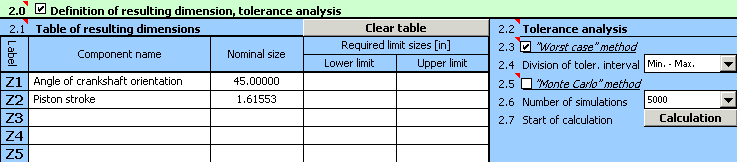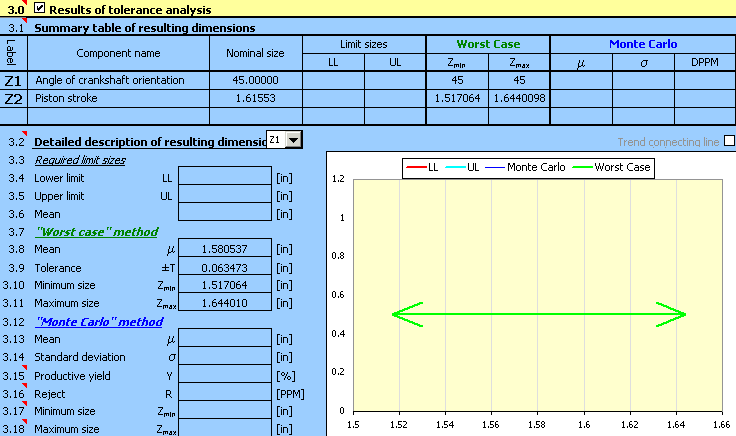#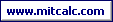Tolerance analysis of the kinematical mechanism using the "Worst Case" method.

For the eccentric crank gear of a piston compressor (see the picture), ascertain the:

1. limit sizes of a piston stroke
2. piston stroke for a random angle of orientation of the driving component (crankshaft)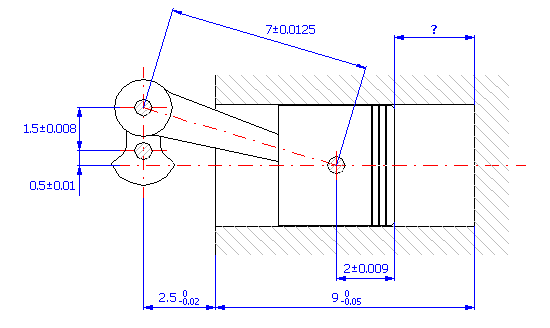If we start from the graphic illustration of the dimensional chain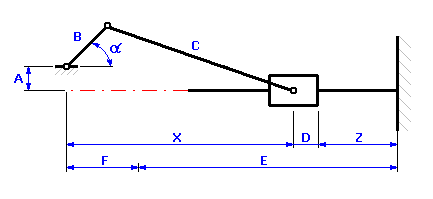we can describe the rate of the piston stroke in the case of the given kinematical mechanism by the relation of: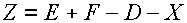where the position of the piston against crankshaft axis X is a kinematical quantity dependent on the angle of orientation of the crankshaft, a. For the eccentric crank gear illustrated in the previous picture, the following relation can be used to set the position of the piston: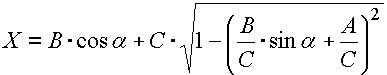The limit positions of the piston against the crankshaft axis are obvious from the following diagram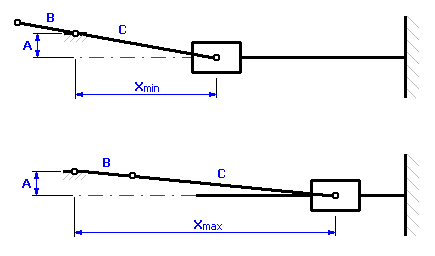and can be described by these relations: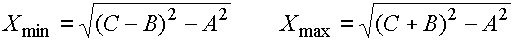### A. Determining the limit values of a piston stroke.

The solution of the task of the tolerance analysis of the kinematical mechanism can be divided into the following steps:

1) In Table [1.1] we will define all the input dimensions (partial components) of the dimensional chain.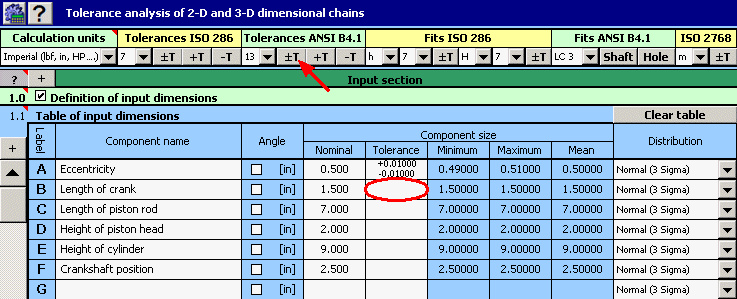2) For the individual dimensions we will further set the rated production tolerances. For dimensions B, C and D, the standardized symmetrical tolerances according to ANSI B4.1 in accuracy level 13 are rated. To set them in the table, we can use the function of automatic tolerance selection.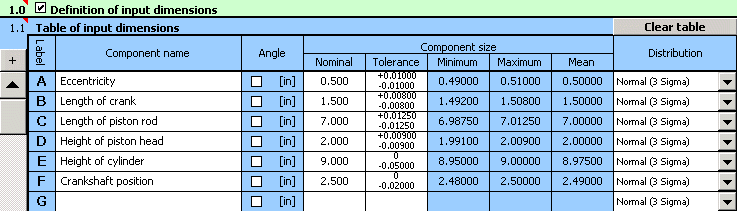3) In Table [2.1] we will define the parameters of the resulting dimensions (closed components) of the dimensional chain. The calculation relations for the minimum and maximum piston stroke defined in the previous paragraph have to be modified into the form (syntax) required by Excel. Therefore, in the second column of the table, we will set the relation "=_E+_F-_D-((_C+_B)^2-_A^2)^0.5" for the minimum piston stroke and the relation "=_E+_F-_D-((_C-_B)^2-_A^2)^0.5" for the maximum piston stroke.

4) In Line [2.3], check the switch next to the "Worst Case" calculation method. In List Box [2.4], further select the "roughest" division of tolerance interval ("Min. - Max." item) which is sufficient for the given task.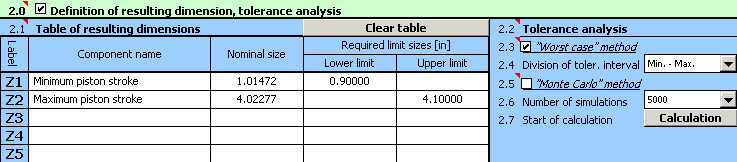5) Press the button on Line [2.7] to run the calculation (tolerance analysis) of the dimensional chain. The limit values of the stroke can be found in Paragraph .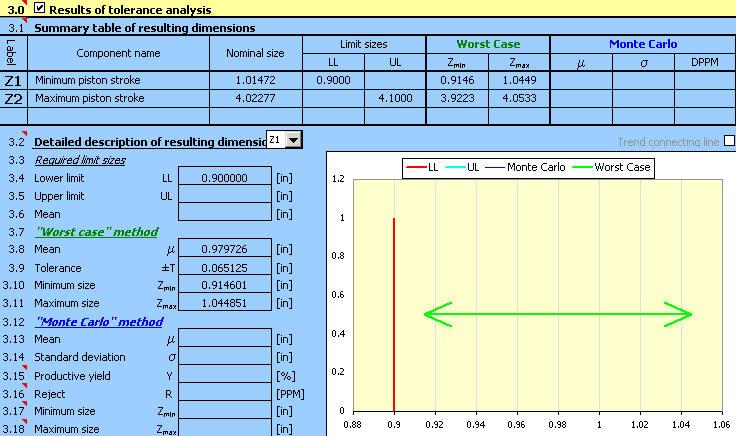### B. Determining the piston stroke for a random angle of crankshaft orientation.

The procedure of solving this task will be similar to the previous one and can be divided into the following steps:

1) The input dimension will be defined in Table [1.1] similarly to example A in steps 1) and 2).2) The angle of crankshaft orientation for which we intend to set the piston stroke will be defined as a constant "Z1" in the first line of Table [2.1]. In the second line of Table "Z2", set the relation for the calculation of the stroke. The above-mentioned calculation relation has to be modified into the form (syntax) required by Excel: